(1) Basic reaction equation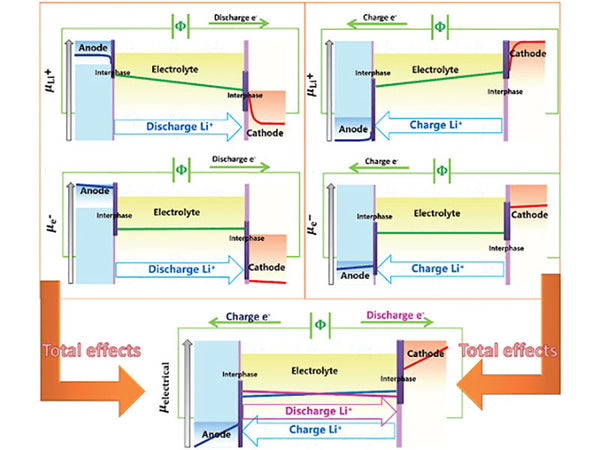The open circuit voltage (OCV) of a lithium ion battery with lithium metal as the negative electrode is expressed as follows:

FE =-{μ (Li, positive electrode)-μ (Li, negative electrode)}

=-{Μ (Li, positive electrode)-μ0 (Li)}

= -2.303RTlg[a(Li, positive electrode)]                        (1-1)

If LiMOn is used as the positive electrode, the battery reaction can be expressed as follows:

Li+(electrolyte)＋e(electrode)＝Li(electrode)                    (1-2)

Li+(electrolyte)＋e(electrode)＋MOn＝LiMOn                   (1-2’)

The lithium chemical potential of the above reaction can be expressed by the following formula:

μ (Li, negative electrode)＋μ0(MOn)==μ0(LiMOn)                   (1-3)(1-3’)

If the change value of Gibbs generation free energy of LiMOn and MOn is obtained, the open circuit voltage (OCV) value can be calculated. Conversely, the Gibbs free energy change of LiMOn can be calculated from the OCV value.

(2) Valence and stabilization energy

With the occurrence of the electrode reaction, the transition metal ion undergoes a redox reaction. For example, the valence state of the transition metal ion in the equation (1-2') changes from 2n+ to (2n-1)+. Therefore, equation (1-3) cannot directly explain the Gibbs free energy change of the reaction. If an intermediate state is designed, the change in the value of the thermodynamic state function can explain this process.

In Figure 1, the three compounds related to the positive electrode reaction, LiMOn and MOn are located in the Li-M-O ternary system triangle, and these three compounds are on a straight line, that is, the Li-MOn pseudo-binary system.Figure 1 - Ternary Li-M-O system (a) Chemical potential diagram of Li-M-O system (b)

In order to give a reasonable explanation from the chemical concept, it is more appropriate to use Li2O and M2O2n-1 as the intermediate states described above. Therefore, the reaction to synthesize LiMOn from Li+MOn can be illustrated by the following hypothetical three-step reaction.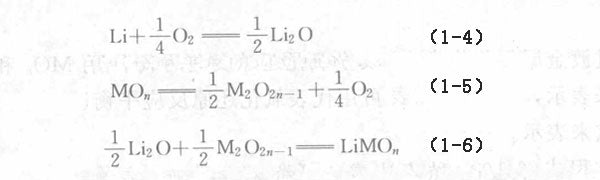The above three reactions are basic chemical reactions. Their thermodynamic processes can be simply described as Li being oxidized, MOn being reduced, and two unit oxides forming binary oxides.

On the other hand, the stability energy of transition metal oxide valence state Δ[M(2n-1)+; M2n+] and binary oxide δ (LiMOn) can be defined as: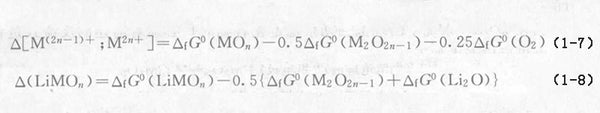Substituting formula (1-7) and formula (1-8) into formula (1-3'), we get: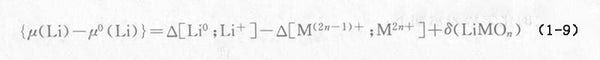The three terms on the right side of the above formula correspond to the three processes of reaction formula (1-4) ~ reaction formula (1-6). Because the first process is the same in any electrode, the positive electromotive force depends on the oxidation of the transition metal and its chemical valence

The reduction process and the interaction between lithium and the transition metal oxide undergoing redox reactions are two factors.

Under normal circumstances, the equilibrium partial pressure of oxygen [p(O2)redox] can be used to characterize the oxidation-reduction reaction of oxides. Using this feature, the effect of the redox reaction on the electromotive force of the electrode can be expressed by the following formula:From the above formula, it seems that the balanced oxygen partial pressure has a greater influence on the electrode electromotive force, but in fact it has no effect on the electrode reaction. However, in the positive electrode reaction, the redox reaction of transition metal ions is always accompanied, and the redox reaction is the characteristic of the electrode reaction.

(3) Chemical potential diagram

The phase relationship of any ternary system shown in Figure 1(a) can be represented by a chemical potential diagram. As mentioned earlier, the equilibrium oxygen partial pressure in the redox reaction plays an important role in determining the electrode potential. The chemical potential diagram of the Li-M-O system can be represented by a coordinate system with the chemical potentials of Li and oxygen as the axis. The schematic diagram of the Li-M-O quasi-binary system is shown in Figure 1(b), and the geometry of Figure 1(b) is related to the thermodynamic properties described in the previous chapter.

When lithium metal is used as a negative electrode, the chemical potential of lithium in the electrode is (Li), and Figure 1(b) is represented by the horizontal line of lithium metal.

The key compound Li2O in equations (1-4) and equations (1-6) is represented as a straight line with a slope of -1/2 in Figure 1(b).

The chemical potential of the positive electrode lithium determined by the equation (1-3) containing the difference in Gibbs energy between LiMOn and MOn is represented by the horizontal line between the stable regions of LiMOn and MOn, and the difference between the two horizontal lines is represented by the FE value.

The equilibrium partial pressure of oxygen at which transition metal oxides undergo redox reactions is represented by the vertical line between MOn and M2O2n-1. μ(Li) can be represented by the intersection of the vertical line representing the equilibrium oxygen partial pressure of the redox reaction and the line representing Li2O.

The first expression of equation (1-10) Δ[Li0; Li+]-Δ[M(2n-1)+; M2n+] represents the poor stability of the valence state when p(O2)=101325Pa.

The interaction corresponds to the vertical width of the LiMOn stable region.

(4) Other factors affecting OCV

When a pseudo-binary stable compound appears in the Li-MO system, the aforementioned reaction formula must be modified. The electrode reaction is considered as follows.

Li (electrolyte) + e (electrode) + M2O2n=LiM2O2n              (1-11)

Li (electrolyte) + e (electrode) + LiM2O2n=2LiMOn             (1-12)

The chemical potential of lithium is expressed as follows:It must be noted that in any electrode reaction, the redox process is always the same, which corresponds to 1 mol of lithium intercalation/deintercalation, and 1 mol of transition metal changes the valence.

When LiM2O2n is stable and does not decompose into LiMOn and M2O2n, the following relationship holds: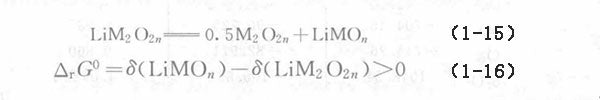From this relationship, we can compare the chemical potential of lithium in three different electrode reactions. As shown in the following relationship, equation (1-13) has the lowest lithium chemical potential.

{μ(Li)-μ0(Li)} (Equation 1-13) < {μ(Li)-μ0(Li)} (Equation 1-3) < {μ(Li)-μ0(Li)} (Equation 1-14)   (1-17)

As the difference between δ(LiMOn) and δ(LiM2O2n) becomes larger, the lithium chemical potential becomes more negative.

When the energy density per mole of lithium is expressed as {μ(Li)-μ0(Li)}dn (dn is the change in the number of lithium measurements), equations (1-3'), equations (1-13), and equations (1-14) indicate that the total energy density of Li to form MOn from LiMOn is a constant and does not depend on whether LiM2O2n is formed. This means that although LiM2O2n has a higher open circuit voltage (OCV), its energy density will not increase.